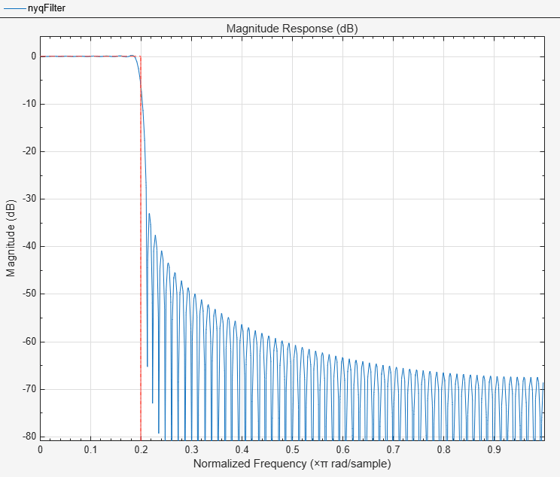# window

FIR filter using windowed impulse response

## Syntax

```h = window(d,fcnhndl,fcnarg,'SystemObject',true) h = window(d,win,'SystemObject',true) ```

## Description

`h = window(d,fcnhndl,fcnarg,'SystemObject',true)` designs a single-rate digital filter System object™ using the specifications in filter specification object `d`.

`fcnhndl` is a handle to a filter design function that returns a window vector, such as the `hamming` or `blackman` functions. `fcnarg` is an optional argument that returns a window. You pass the function to `window`.

`h = window(d,win,'SystemObject',true)` designs a filter using the vector you supply in `win`. The length of vector `win` must be the same as the impulse response of the filter, which is equal to the filter order plus one.

## Examples

collapse all

This example designs a filter using the two design techniques of specifying a function handle and passing a window vector as an input argument.

Use a window vector provided by the kaiser window function to design a Nyquist filter. The window length must be the filter order plus one.

```d = fdesign.nyquist(5,'n',150); % Kaiser window with beta parameter 2.5 nyqFilter = window(d,'window',kaiser(151,2.5),'SystemObject',true); fvtool(nyqFilter)```